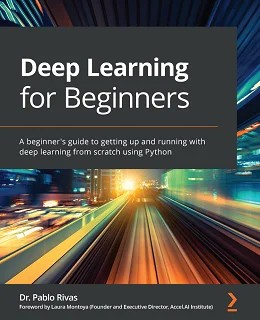# Deep Learning for Beginners### eBook Details:

• Paperback: 376 pages
• Publisher: WOW! eBook (October 9, 2020)
• Language: English
• ISBN-10: 1838640851
• ISBN-13: 978-1838640859

### eBook Description:

Deep Learning for Beginners: Implementing supervised, unsupervised, and generative deep learning (DL) models using Keras, TensorFlow, and PyTorch

With information on the web exponentially increasing, it has become more difficult than ever to navigate through everything to find reliable content that will help you get started with deep learning (DL). This book is designed to help you if you’re a beginner looking to work on deep learning and build deep learning models from scratch, and already have the basic mathematical and programming knowledge required to get started.

The book begins with a basic overview of machine learning, guiding you through setting up popular Python frameworks. You will also understand how to prepare data by cleaning and preprocessing it for deep learning, and gradually go on to explore neural networks. A dedicated section will give you insights into the working of neural networks by helping you get hands-on with training single and multiple layers of neurons. Later, you will cover popular neural network architectures such as CNNs, RNNs, AEs, VAEs, and GANs with the help of simple examples and even build models from scratch. At the end of each chapter, you will find a question and answer section to help you test what you’ve learned through the course of the book.

• Implement recurrent neural networks (RNNs) and long short-term memory networks (LSTMs) in image classification and NLP
• Understand the mathematical terminology associated with DL algorithms
• Explore the role of convolutional neural networks (CNNs) in computer vision and signal processing
• Understand the ethical implications of DL modeling
• Code a generative adversarial network (GAN) and a variational autoencoder (VAE) to generate images from a learned latent space
• Implement visualization techniques to compare deep and variational autoencoders

By the end of this Deep Learning for Beginners book, you’ll be well-versed with deep learning concepts and have the knowledge you need to use specific algorithms with various tools for different tasks.

### 4 Responses

1. March 2, 2021

[…] Codeless Deep Learning with KNIME: Discover how to integrate KNIME Analytics Platform with deep learning libraries to implement artificial intelligence solutions […]

2. June 24, 2021

[…] Deep Learning from the Basics begins with a fast-paced introduction to deep learning with Python, its definition, characteristics, and applications. You’ll learn how to use the Python interpreter and the script files in your applications, and utilize NumPy and Matplotlib in your deep learning models. As you progress through the book, you’ll discover backpropagation – an efficient way to calculate the gradients of weight parameters – and study multilayer perceptrons and their limitations, before, finally, implementing a three-layer neural network and calculating multidimensional arrays. […]

3. June 24, 2021

[…] Deep Learning from the Basics begins with a fast-paced introduction to deep learning with Python, its definition, characteristics, and applications. You’ll learn how to use the Python interpreter and the script files in your applications, and utilize NumPy and Matplotlib in your deep learning models. As you progress through the book, you’ll discover backpropagation – an efficient way to calculate the gradients of weight parameters – and study multilayer perceptrons and their limitations, before, finally, implementing a three-layer neural network and calculating multidimensional arrays. […]

4. June 24, 2021

[…] Deep Learning from the Basics begins with a fast-paced introduction to deep learning with Python, its definition, characteristics, and applications. You’ll learn how to use the Python interpreter and the script files in your applications, and utilize NumPy and Matplotlib in your deep learning models. As you progress through the book, you’ll discover backpropagation – an efficient way to calculate the gradients of weight parameters – and study multilayer perceptrons and their limitations, before, finally, implementing a three-layer neural network and calculating multidimensional arrays. […]